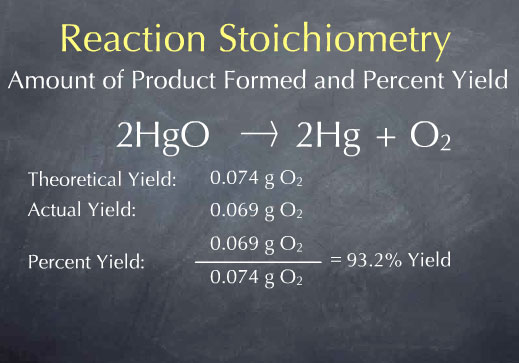# If the reaction of 0.112 grams of H2 produces 0.745 grams of H2O, what is the percent yield? Fe3O4+4H2=>3Fe+4H2O

Sep 18, 2014

The percent yield is 74.4 %.

Fe₃O₄ + 4H₂ → 3Fe + 4H₂O

First, calculate the theoretical yield of H₂O.

Theor. yield = $\text{0.112 g H"_2 × ("1 mol H"_2)/("2.016 g H"_2) × ("4 mol H"_2"O")/("4 mol H"_2) × ("18.02 g H"_2"O")/("1 mol H"_2"O") = "1.001 g H"_2"O}$

Now calculate the percent yield.

% yield = "actual yield"/"theoretical yield" × 100 % = "0.745 g"/"1.001 g" × 100 % = 74.4 %